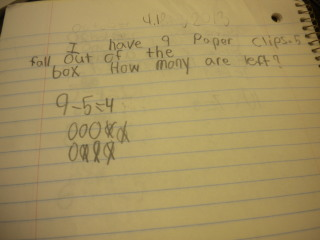# Counting Back

15 teachers like this lesson
Print Lesson

## Objective

SWBAT use counting back as a strategy to find the difference in single digit numbers.

#### Big Idea

Smack down! In this lesson students will learn how to count back to subtract.

## Activating Strategy

10 minutes

Counting back from a number is a strategy that builds children’s mental math skills.  Children begin to identify patterns as they count back, allowing them to understand the relationship between the numbers they are counting.  This lays a foundation for adding and subtracting greater numbers and recognizing patterns between numbers within 100.  One way for children to remember where to start counting back is through concrete examples.

In first grade students realize that doing mathematics involves solving problems and discussing how they solved them.  Getting the student to look at the answer ans ask themselves "does this make sense?" is a key to MP1.

To begin the lesson, have children practice counting forward and backward from any number using counters.  Give them directions such as the following:

• Start with 4.  Count forward.  What are the next 3 numbers? (5, 6, 7)
• Start with 8.  Count backward.  What are the next 2 numbers? (7, 6)
• Start with 10.  Count forward.  What are the next 3 numbers? (11, 12, 13)

Challenge volunteers to give counting directions for the rest of the class to follow.

## Teaching Strategies

15 minutes

I start by writing the following problem on the board or chart paper (a PPT is also available count back)

How can you count back to find the difference for 9 – 1?

I guide the discussion using the following questioning:

• How do you know where to start counting back? (I start at 9, because it is the number that I am subtracting from.)
• How can we use counters to solve 9 – 1? (I can count back one counter to show the number that I subtract.  The number I stop on is the difference.)

I tell the students they used counting back to find the difference.  Then read the following problem:

How can you count back to find the difference for 9 – 2?

How can you count back to find the difference for 8 – 1 ?

I have children find the difference by using the counters to count back.  Then I have children record the difference.  I use similar questioning for 9 – 3.  I try to focus on children’s understanding of counting back 1, 2, or 3 to subtract.  I have children count back from 10 to 1 to help them learn the pattern.

## Independent Practice

30 minutes

For the independent practice on this lesson, I use count back worksheet to allow students the opportunities to problem solve independently.

To extend the thinking, challenge children to find a way to check their answers.  For example invite volunteers to share their methods with the class.  If no one uses addition to check the answer, you might ask the children to figure out a way to undo counting back by counting on.

For those students who are struggling, I like to use more concrete models to show them how we use counting back as a subtraction strategy.  Write 12 – 3 = _____ on a sentence strip or personal white board.  Tell the children to place 12 counters in a row to represent the starting number.  Have them turn the counters so that they are all on the red side.  Guide the discussion as follows:

• How many counters do you need to count back from 12?  (3)

Tell children to count back 3 counters by turning over each counter as they count back 3.

• How many yellow counters are there?  (3 yellow counters)
• How many red counters are there?  (9 red counters)
• What is 12 – 3? (9)

Continue using the same technique asking students to find several other counting back differences.

In this video, one of my struggling students still had difficulty with counting back, so he continued to use counters to subtract.

## Closing/Summarizing

5 minutes

To close out the lesson, I have students answer the following in their math journal:

I have 9 paperclips.  5 paperclips fall out of the box.  How many are left?

This is a sample of a student journal for this lesson:I like to use journals as a quick check for understanding.  This also allows the opportunity for students to write about their learning.